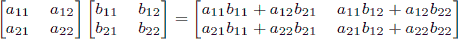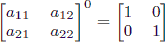문제1620--FibonacciNumber

### 1620: FibonacciNumber

실행시간 제한: 1 Sec  메모리사용 제한: 128 MB
제출: 104  통과: 33
[제출] [채점기록] [묻고답하기]

#### 문제 설명

f(0)=0, f(1)=1, f(i)=f(i-1)+f(i-2) if i>=2.

Calculate f(n) % 1000000007.

#### 입력 설명

The first line of the input gives the number of test cases, T (1 <= T <= 200).

For each test case integer n, which will be between 0 and 1,000,000,000, is given.

#### 출력 설명

For each test case, print f(n) modular 1,000,000,007 as explained in the problem statement, in one line.

#### 입력 예시 Copy

4
0
1
10
50

#### 출력 예시 Copy

0
1
55
586268941

#### 도움

As a reminder, matrix multiplication is associative, and the product of two 2 × 2 matrices is given byAlso, note that raising any 2 × 2 matrix to the 0th power gives the identity matrix: